Open in App
Not now

# ML | Normal Equation in Linear Regression

• Difficulty Level : Medium
• Last Updated : 11 Jan, 2023

Normal Equation is an analytical approach to Linear Regression with a Least Square Cost Function. We can directly find out the value of θ without using Gradient Descent. Following this approach is an effective and time-saving option when working with a dataset with small features. Normal Equation method is based on the mathematical concept of Maxima & Minima in which the derivative and partial derivative of any function would be zero at the minima and maxima point. So, in Normal Equation method, we get the minimum value of the Cost function by finding its partial derivative w.r.t to each weight and equating it to zero.

The normal Equation is as follows: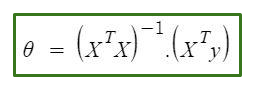In the above equation,

θ: hypothesis parameters that define it the best.
X: Input feature value of each instance.
Y: Output value of each instance.

### Maths Behind the equation:

Given the hypothesis function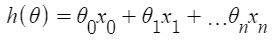where,
n: the no. of features in the data set.
x0: 1 (for vector multiplication)
Notice that this is a dot product between θ and x values. So for the convenience to solve we can write it as: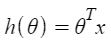The motive in Linear Regression is to minimize the cost function

J(\Theta) = \frac{1}{2m} \sum_{i = 1}^{m} \frac{1}{2} [h_{\Theta}(x^{(i)}) – y^{(i)}]^{2}

where,
xi: the input value of iih training example.
m: no. of training instances
n: no. of data-set features
yi: the expected result of ith instance

Let us represent the cost function in a vector form.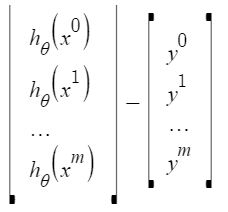We have ignored 1/2m here as it will not make any difference in the working. It was used for mathematical convenience while calculating gradient descent. But it is no more needed here.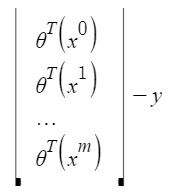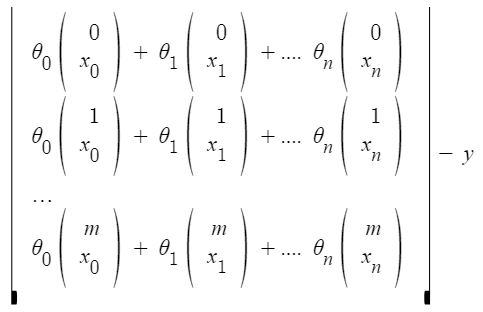xij: value of jih feature in iih training example.

This can further be reduced toBut each residual value is squared. We cannot simply square the above expression. As the square of a vector/matrix is not equal to the square of each of its values. So to get the squared value, multiply the vector/matrix with its transpose. So, the final equation derived is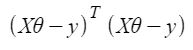Therefore, the cost function is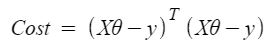So, now getting the value of θ using the partial derivative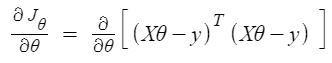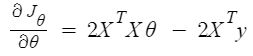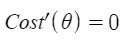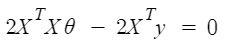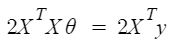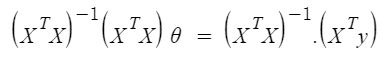So, this is the finally derived Normal Equation with θ giving the minimum cost value.

Example:

## Python3

 # This code may not run on GFG IDE  # as required modules not found.     # import required modules  import numpy as np  import matplotlib.pyplot as plt  from sklearn.datasets import make_regression     # Create data set.  x, y = make_regression(n_samples=100, n_features=1,                         n_informative=1, noise=10, random_state=10)     # Plot the generated data set.  plt.scatter(x, y, s=30, marker='o')  plt.xlabel("Feature_1 --->")  plt.ylabel("Target_Variable --->")  plt.title('Simple Linear Regression')  plt.show()     # Convert  target variable array from 1d to 2d.  y = y.reshape(100, 1)

Output: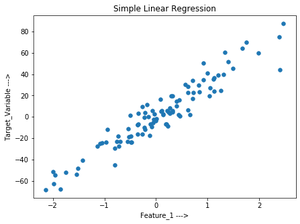Let’s implement  the Normal Equation:

## Python3

 # code     # Adding x0=1 to each instance  x_new = np.array([np.ones(len(x)), x.flatten()]).T     # Using Normal Equation.  theta_best_values = np.linalg.inv(x_new.T.dot(x_new)).dot(x_new.T).dot(y)     # Display best values obtained.  print(theta_best_values)

[[ 0.52804151]
[30.65896337]]

Try to predict for new data instance:

## Python3

 # sample data instance.  x_sample = np.array([[-2], ])     # Adding x0=1 to each instance.  x_sample_new = np.array([np.ones(len(x_sample)), x_sample.flatten()]).T     # Display the sample.  print("Before adding x0:\n", x_sample)  print("After adding x0:\n", x_sample_new)

Before adding x0:
[[-2]
[ 4]]
[[ 1. -2.]
[ 1.  4.]]

## Python3

 # code  # predict the values for given data instance.  predict_value=x_sample_new.dot(theta_best_values)  print(predict_value)

[[-60.78988524]
[123.16389501]]

## Python3

 # code     # Plot the output.  plt.scatter(x,y,s=30,marker='o')  plt.plot(x_sample,predict_value,c='red')  plt.plot()  plt.xlabel("Feature_1 --->")  plt.ylabel("Target_Variable --->")  plt.title('Simple Linear Regression')  plt.show()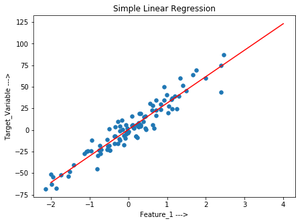Verify the above using sklearn LinearRegression class:

## Python3

 # code     # Verification.  from sklearn.linear_model import LinearRegression  lr=LinearRegression()    # Object.  lr.fit(x,y)              # fit method.     # Print obtained theta values.  print("Best value of theta:",lr.intercept_,lr.coef_,sep='\n')     #predict.  print("predicted value:",lr.predict(x_sample),sep='\n')

Best value of theta:
[0.52804151]
[[30.65896337]]

predicted value:
[[-60.78988524]
[123.16389501]]

My Personal Notes arrow_drop_up
Related Articles